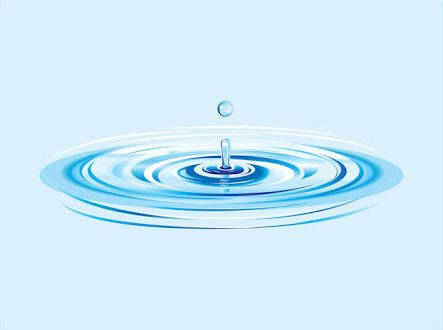# Water Budget

## Components of Water Budget

The water budget of a unit area of the earth’s surface is formed from the components:

P = precipitation

E = Evaporation

D = Discharge, Runoff

R = Reserve, storage and

U = Use, consumption

• The conservation law for water satisfies the water balance.

P = E + D + R + U

This equation states that, water added to the earth’s surface by precipitation is partitioned among the components E, D, R and U.

R is a temporary storage of water in the soils and U designates chemically or physically bound water.

It can be assumed that R and U are constant, and so fluctuations of these quantities bcame insignificant in the water balance.

• Therefore, the equation can e simplified to

P = E + D

From continuity principles it can be assumed that, for the entire earth, the quantity of water that evaporates from the surface is equal to the quantity that is replaced as precipitation. For the whole earth (G = Global) therefore, it has to be true:

PG = EG

This does not mean that the components, P, E and D are equal on land and water surfaces; they are much different. If one estimates for land (L) or ocean (S) surfaces the quanitites of precipitation PL , PS and Evaporation EL , Eit can be shown that PL ≠ EL and PS ≠ ES.

The inequality causes the flux of water vapor from the ocean surfaces to the continents  (DS), and the runoff from the land surfaces to the oceans (DL), i.e. is responsible to the circulation of water among earth zones and among continents and oceans.

The balance is defined by PZ = EZ only for internally drained regions of the continents (Z), where DZ = 0

Over the oceans, total evaporation exceeds the total precipitation. The difference-

Ds = Es – Ps

Is compensated by the runoff from the continents-

DL = PL – EL

Where, on the average, precipitation exceeds evaporation.

## Concluding Remarks

• Most forms of life get supplies of critical nutrients from compounds containing carbon, nitrogen, phosphorus, and sulfur that are circulated in their respective chemical cycles.
• These cycles are driven by gravity and by the one-way flow of energy from the sun. Human activities are degrading the earth’s natural capital by increasingly altering the rates at which energy flows through these cycles and the rates at which critical chemicals are recycled.

This is a major intrusion into the processes of energy flow and nutrient cycling that support life on the earth.

Article RatingCurrently studying at Department of Botany, University of Dhaka. E-mail: tareksiddikitaki@gmail.com Minimum monthly resolution- publish (1), revise (2), share (2)

## Salinity: Sources, Cycles & Classification

Over four-fifths of the surface of our planet is covered with a salt solution (oceans …

Subscribe
Notify of